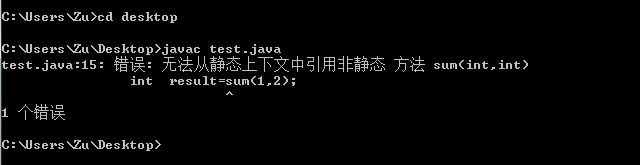# 静态方法与非静态方法的区别

首先，两者本质上的区别是：静态方法是在类中使用staitc修饰的方法，在类定义的时候已经被装载和分配。而非静态方法是不加static关键字的方法，在类定义时没有占用内存，只有在类被实例化成对象时，对象调用该方法才被分配内存。

其次，静态方法中只能调用静态成员或者方法，不能调用非静态方法或者非静态成员，而非静态方法既可以调用静态成员或者方法又可以调用其他的非静态成员或者方法。

class Test{
public int sum(int a,int b){//非静态方法
return a+b;
}
public static void main(String[] args){
int  result=sum(1,2);//静态方法调用非静态方法
System.out.println("result="+result);
}
}class Test{
public static int sum(int a,int b){//加入static关键字，变成静态方法
return a+b;
}
public static void main(String[] args){
int  result=sum(1,2);//静态方法调用静态方法
System.out.println("result="+result);
}
}

class Test{
public int sum(int a,int b){
return a+b;
}
public static void main(String[] args){
Test test=new Test();//实例化类
int  result=test.sum(1,2);//调用非静态方法
System.out.println("result="+result);
}
}